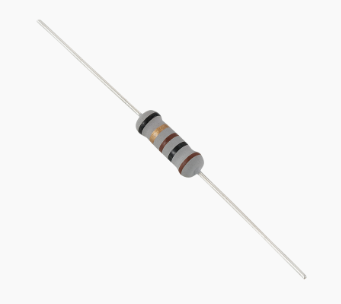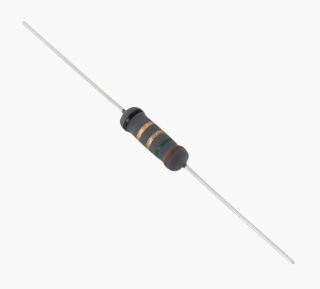What Is A Fusible Resistor : Working Principle & Its Applications
Aug. 10, 2022

A fusible resistor known by the name current-limiting resistor, fuse resistor, meltdown resistor is a type of resistor that has a low power rating and is designed to melt when a certain amount of current flows through it, thus breaking an electrical circuit. It is the only type of resistor designed to quickly burn up (and isolate itself) when overloaded with the current. Fusible resistors are used as safety devices to prevent electrical fires and other damage caused by excessive current being applied at once. They can be found in household appliances like washing machines and microwaves, as well as electronic devices like stereos. To learn more about fusible resistors and how they work, check out our below article.## What is a Fusible Resistor / Definition?

Fusible Resistors are power resistors that are designed to fail in a controlled manner. These resistors start as high-power resistors, then have their lead ends welded together and placed in a ceramic enclosure. If the resistor has too much current applied to it, it will short out and the resulting heat will cause the fusible resistor to melt, encapsulating the melted material.

Because of their design, they can only be used once, but they can be used in applications where they may need to be replaced because they are intended to fail.

## Fusible Resistor Construction

The resistor consists of a non-inductive ceramic core with a winding of high resistance wire. The core is coated with a special glaze and the entire assembly is enclosed in a tough non-hygroscopic resin or epoxy case.

Fusible resistors are constructed such that their resistance increases as the temperature rises. This characteristic allows the resistor to function as both a current limiting device and as an over-current fuse, protecting circuits from transient over-current surges and fault conditions.

There are two most commonly used ways to construct a fusible resistor which are as follows

ㆍThe first is a series of high resistance metal films sandwiched between two end caps, which are connected to the ends of the films.

ㆍThe second consists of a non-metallic element wound into a coil and then coated with a thin layer of metal.

## Fusible Resistor Circuit

The main function of a fusible resistor is to restrict the inrush current flow. So this resistor is used as the overcurrent component within the charger or power supply circuit that can have 10ohms of resistance. This circuit is used to protect over current & restrict inrush current.

In contrast, a fuse and an NTC thermistor can also be used to attain inrush current limitation & equivalent over current protection. But by using Fusible resistors, we can save space instead of using separate thermistors & fuses. But, the heat produced through a fusible resistor could need spacing in between components. When this resistor is rated up to 10 then other parts need 0.5-inch spacing. Similarly, if this resistor is rated above 10 W then 1-inch space is necessary.

When power supplies, as well as chargers, need to be in small, then compact packages & the necessary spacing in the region of the fusible resistors could leave the designer of a circuit with insufficient space for the whole design.## Fusible Resistor Size Calculation

Calculating a fusible resistor value is a bit different from calculating other types of resistors. Here’s how:

Calculate the resistance you’ll need by taking into account the voltage and current of the circuit in question.

Find the appropriate power rating for your resistor, based on whether it will be in series or parallel with your circuit. This can be done by multiplying voltage by current and then dividing by 2 (for series) or 1 (for parallel).

Choose a fusible resistor that has a power rating as close as possible to your intended power rating, without being higher than it.

Determine the maximum current that will flow through it. You can calculate this using Ohm’s law which states that V = I x R, where V is voltage, I is current, and R is resistance.

For example, if your voltage is 12 volts and your desired resistance is 8 ohms, then the current through your fusible resistor would be 1.5 amps (V = 12 volts; R = 8 ohms; I = V/R = 1.5 amps).

## Applications/How Are Fusible Resistors Used?

The applications of fusible resistors include the following.

ㆍFusible resistors are used in electrical circuits that may occasionally be exposed to short circuit conditions for a short period of time. They will blow out like a fuse in the event of a short circuit, protecting the circuit from damage and fire.

ㆍFusible resistors are used in motor controller systems, automobiles, power supplies, rectifiers and inverters, home appliances, power tools, and other equipment.

ㆍThe main benefit of using this resistor is that its resistance function controls inrush current.

ㆍThis resistor is used as the main above current component within a charger or a power supply.

ㆍA fusible resistor performs dual functions. Once the power supply is exceeded, then it works like a resistor limiting current. Similarly, once the rating of power is exceeded, then it works as a fuse in the circuit to defend different components within the circuit from the surplus current.

## Difference between A Fuse And A Fusible Resistor

A fusible resistor acts as a safety fuse, but there is a difference between a fuse and a fusible resistor.

A normal glass tube fuse used where high surge current load devices used like 2A, 8A, 10A, etc and this will burn when high load occurs according to fuse capacity or when short connection.

The fusible resistor used in the circuit operates high voltage with small or lower currents such as 100ma, 250ma. A fusible resistor can pass the pulse of a high volt like 500v without failure, but it must fuse quickly without flames when 100v short circuit overloads.